Functions and CALL Routines

# Using Random-Number Functions and CALL Routines

Two types of random-number functions are available in SAS. The newest random-number function is the RAND function. It uses the Mersenne-Twister pseudo-random number generator (RNG) that was developed by Matsumoto and Nishimura (1998). This RNG has a very long period of 219937 - 1, and has very good statistical properties. (A period is the number of occurrences before the pseudo-random number sequence repeats.)

The RAND function is started with a single seed. However, the state of the process cannot be captured by a single seed, which means that you cannot stop and restart the generator from its stopping point. Use the STREAMINIT function to produce a sequence of values that begins at the beginning of a stream. For more information, see the Details section of the RAND Function.

The older random-number generators include the UNIFORM, NORMAL, RANUNI, RANNOR, and other functions that begin with RAN. These functions have a period of only 231 - 2 or less. The pseudo-random number stream is started with a single seed, and the state of the process can be captured in a new seed. This means that you can stop and restart the generator from its stopping point by providing the proper seed to the corresponding CALL routines. You can use the random-number functions to produce a sequence of values that begins in the middle of a stream.

Random-number functions and CALL routines generate streams of pseudo-random numbers from an initial starting point, called a seed, that either the user or the computer clock supplies. A seed must be a nonnegative integer with a value less than 231-1 (or 2,147,483,647). If you use a positive seed, you can always replicate the stream of random numbers by using the same DATA step. If you use zero as the seed, the computer clock initializes the stream, and the stream of random numbers cannot be replicated.

### Using the DATA Step to Generate a Single Stream of Random Numbers

The DATA steps in this section illustrate several properties of the random-number functions. Each of the DATA steps that call a function generates a single stream of pseudo-random numbers based on a seed value of 7, because that is the first seed for the first call for every step. Some of the DATA steps change the seed value in various ways. Some of the steps have single function calls and others have multiple function calls. None of these DATA steps change the seed. The only seed that is relevant to the function calls is the seed that was used with the first execution of the first random-number function. There is no way to create separate streams with functions (CALL routines are used for this purpose), and the only way you can restart the function random-number stream is to start a new DATA step.

The following example executes multiple DATA steps:

```options nodate pageno=1 linesize=80 pagesize=60;

/* This DATA step produces a single stream of random numbers */
/* based on a seed value of 7.                               */
data a;
a = ranuni (7); output;
a = ranuni (7); output;
a = ranuni (7); output;
a = ranuni (7); output;
a = ranuni (7); output;
a = ranuni (7); output;
a = ranuni (7); output;
a = ranuni (7); output;
a = ranuni (7); output;
a = ranuni (7); output;
a = ranuni (7); output;
a = ranuni (7); output;
run;

/* This DATA step uses a DO statement to produce a single */
/* stream of random numbers based on a seed value of 7.   */
data b (drop = i);
do i = 7 to 18;
b = ranuni (i);
output;
end;
run;

/* This DATA step uses a DO statement to produce a single */
/* stream of random numbers based on a seed value of 7.   */
data c (drop = i);
do i = 1 to 12;
c = ranuni (7);
output;
end;
run;

/* This DATA step calls the RANUNI and the RANNOR functions */
/* and produces a single stream of random numbers based on  */
/* a seed value of 7.                                       */
data d;
d = ranuni (7); f = ' '; output;
d = ranuni (8); f = ' '; output;
d = rannor (9); f = 'n'; output;
d = .;          f = ' '; output;
d = ranuni (0); f = ' '; output;
d = ranuni (1); f = ' '; output;
d = rannor (2); f = 'n'; output;
d = .;          f = ' '; output;
d = ranuni (3); f = ' '; output;
d = ranuni (4); f = ' '; output;
d = rannor (5); f = 'n'; output;
d = .;          f = ' '; output;
run;

/* This DATA step calls the RANNOR function and produces a     */
/* single stream of random numbers based on a seed value of 7. */
data e (drop = i);
do i = 1 to 6;
e = rannor (7); output;
e = .;          output;
end;
run;

/* This DATA step merges the output data sets that were */
/* created from the previous five DATA steps.           */
data five;
merge a b c d e;
run;

/* This procedure writes the output from the merged data sets. */
proc print label data=five;
options missing = ' ';
label f = '00'x;
title 'Single Random Number Streams';
run;```

The following output shows the program results.

```                          Single Random Number Streams                         1

Obs       a          b          c           d                e

1    0.29474    0.29474    0.29474     0.29474          0.39464
2    0.79062    0.79062    0.79062     0.79062
3    0.79877    0.79877    0.79877     0.26928    n     0.26928
4    0.81579    0.81579    0.81579
5    0.45122    0.45122    0.45122     0.45122          0.27475
6    0.78494    0.78494    0.78494     0.78494
7    0.80085    0.80085    0.80085    -0.11729    n    -0.11729
8    0.72184    0.72184    0.72184
9    0.34856    0.34856    0.34856     0.34856         -1.41879
10    0.46597    0.46597    0.46597     0.46597
11    0.73523    0.73523    0.73523    -0.39033    n    -0.39033
12    0.66709    0.66709    0.66709```

The pseudo-random number streams in output data sets A, B, and C are identical. The stream in output data set D mixes calls to the RANUNI and the RANNOR functions. In observations 1, 2, 5, 6, 9, and 10, the values that are returned by RANUNI exactly match the values in the previous streams. Observations 3, 7, and 11, which are flagged by "n", contain the values that are returned by the RANNOR function. The mix of the function calls does not affect the generation of the pseudo-random number stream. All of the results are based on a single stream of uniformly distributed values, some of which are transformed and returned from other functions such as RANNOR. The results of the RANNOR function are produced from two internal calls to RANUNI. The DATA step that creates output data set D executes the following steps three times to create 12 observations:

• call to RANUNI

• call to RANUNI

• call to RANNOR (which internally calls RANUNI twice)

• skipped line to compensate for the second internal call to RANUNI

In the DATA step that creates data set E, RANNOR is called six times, each time skipping a line to compensate for the fact that two internal calls to RANUNI are made for each call to RANNOR. Notice that the three values that are returned from RANNOR in the DATA step that creates data set D match the corresponding values in data set E.

### Using the %SYSFUNC Macro to Generate a Single Stream of Random Numbers

When the RANUNI function is called through the macro language by using %SYSFUNC, one pseudo-random number stream is created. You cannot change the seed value unless you close SAS and start a new SAS session. The %SYSFUNC macro produces the same pseudo-random number stream as the DATA steps that generated the data sets A, B, and C for the first macro invocation only. Any subsequent macro calls produce a continuation of the single stream.

```%macro ran;
%do i = 1 %to 12;
%let x = %sysfunc (ranuni (7));
%put &x;
%end;
%mend;

%ran;```

SAS writes the following output to the log:

```10   %macro ran;
11      %do i = 1 %to 12;
12         %let x = %sysfunc (ranuni (7));
13         %put &x;
14      %end;
15   %mend;
16   %ran;
0.29473798875451
0.79062100955779
0.79877014262544
0.81579051763554
0.45121804506109
0.78494144826426
0.80085421204606
0.72184205973606
0.34855818345609
0.46596586120592
0.73522999404707
0.66709365028287```

Each random-number function and CALL routine generates pseudo-random numbers from a specific statistical distribution. Each random-number function requires a seed value expressed as an integer constant or a variable that contains the integer constant. Each CALL routine calls a variable that contains the seed value. Additionally, every CALL routine requires a variable that contains the generated pseudo-random numbers.

The seed variable must be initialized before the first execution of the function or CALL routine. After each execution of a function, the current seed is updated internally, but the value of the seed argument remains unchanged. However, after each iteration of the CALL routine the seed variable contains the current seed in the stream that generates the next pseudo-random number. With a function, it is not possible to control the seed values, and, therefore, the pseudo-random numbers after the initialization.

Except for the NORMAL and UNIFORM functions, which are equivalent to the RANNOR and RANUNI functions, respectively, SAS provides a CALL routine that has the same name as each random-number function. Using CALL routines gives you greater control over the seed values.

### Overview of Random-Number CALL Routines and Streams

You can use the random-number CALL routines to generate multiple streams of pseudo-random numbers within a single DATA step. If you supply a different seed value to initialize each of the seed variables, the streams of the generated pseudo-random numbers are computationally independent, but they might not be statistically independent unless you select the seed values carefully.

Note:   Although you can create multiple streams with multiple seeds, this practice is not recommended. It is always safer to create a single stream. With multiple streams, as the streams become longer, the chances of the stream overlapping increase.The following two examples deliberately select seeds to illustrate worst-case scenarios. The examples show how to produce multiple streams by using multiple seeds. Although this practice is not recommended, you can use the random-number CALL routines with multiple seeds.

### Example 1: Using Multiple Seeds to Generate Multiple Streams

This example shows that you can use multiple seeds to generate multiple streams of pseudo-randomly distributed values by using the random-number CALL routines. The first DATA step creates a data set with three variables that are normally distributed. The second DATA step creates variables that are uniformly distributed. The SGSCATTER procedure (see the SAS ODS Graphics: Procedures Guide) is used to show the relationship between each pair of variables for each of the two distributions.

```options pageno = 1 nodate ls = 80 ps = 64;

data normal;
seed1 = 11111;
seed2 = 22222;
seed3 = 33333;
do i = 1 to 10000;
call rannor(seed1, x1);
call rannor(seed2, x2);
call rannor(seed3, x3);
output;
end;
run;

data uniform;
seed1 = 11111;
seed2 = 22222;
seed3 = 33333;
do i = 1 to 10000;
call ranuni(seed1, x1);
call ranuni(seed2, x2);
call ranuni(seed3, x3);
output;
end;
run;

proc sgscatter data = normal;
title 'Nonindependent Random Normal Variables';
plot x1*x2 x1*x3 x3*x2 / markerattrs = (size = 1);
run;

proc sgscatter data = uniform;
title 'Nonindependent Random Uniform Variables';
plot x1*x2 x1*x3 x3*x2 / markerattrs = (size = 1);
run;```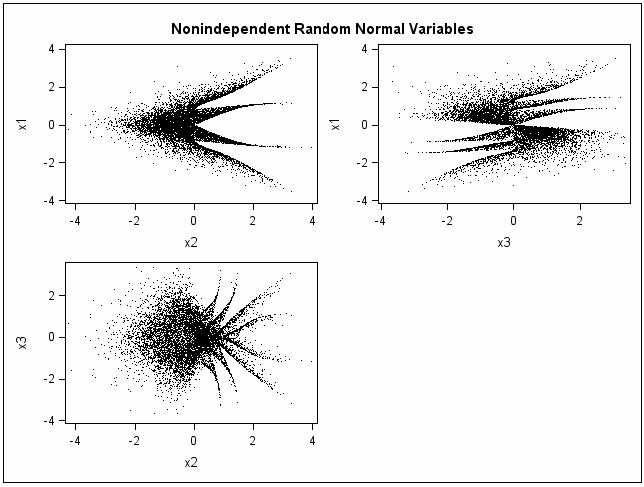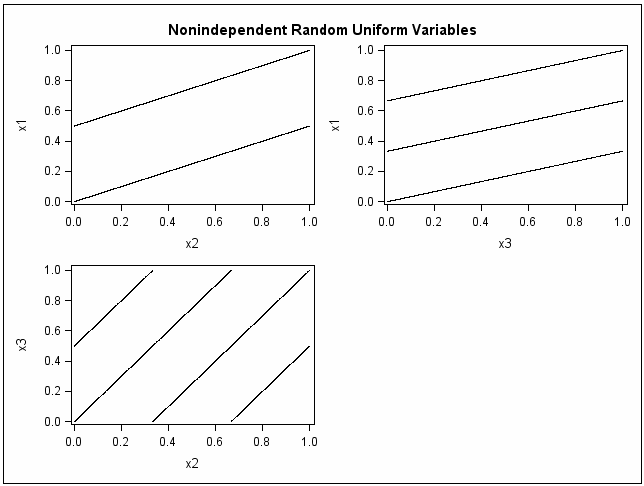The first plot (Multiple Streams from Multiple Seeds: Nonindependent Random Normal Variables) shows that normal variables appear to be linearly uncorrelated, but they are obviously not independent. The second plot (Multiple Streams from Multiple Seeds: Nonindependent Random Uniform Variables) shows that uniform variables are clearly related. With this class of random-number generators, there is never any guarantee that the streams will be independent.

### Example 2: Using Different Seeds with the CALL RANUNI Routine

The following example uses three different seeds and the CALL RANUNI routine to produce multiple streams.

```data uniform(drop=i);
seed1 = 255793849;
seed2 =1408147117;
seed3 = 961782675;
do i=1 to 10000;
call ranuni(seed1, x1);
call ranuni(seed2, x2);
call ranuni(seed3, x3);
i2 = lag(x2);
i3 = lag2(x3);
output;
end;
label i2='Lag(x2)' i3='Lag2(x3)';
run;

title 'Random Uniform Variables with Overlapping Streams';
proc sgscatter data=uniform;
plot x1*x2 x1*x3 x3*x2 / markerattrs = (size = 1);
run;

proc sgscatter data=uniform;
plot i2*x1 i3*x1 / markerattrs = (size = 1);
run;

proc print noobs data=uniform(obs=10);
run;```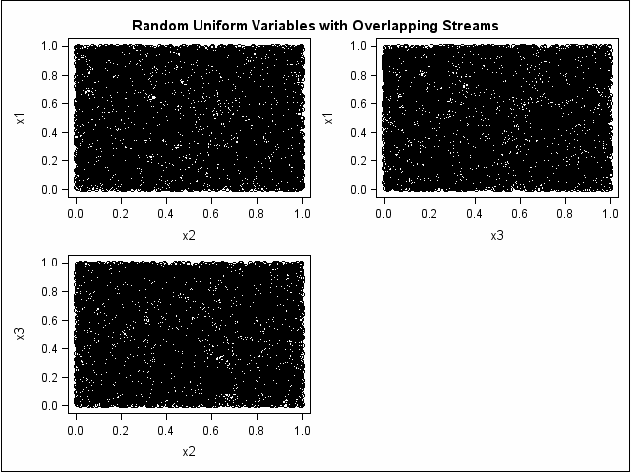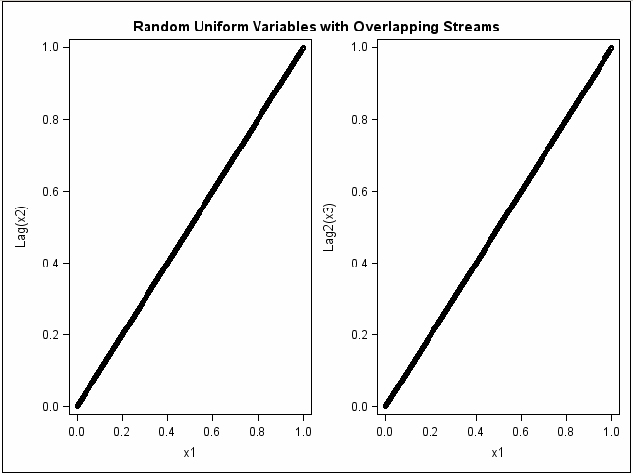```               Random Uniform Variables with Overlapping Streams               2

seed1       seed2       seed3       x1       x2       x3       i2       i3

1408147117   961782675   383001085  0.65572  0.44786  0.17835   .        .
961782675   383001085  1989090982  0.44786  0.17835  0.92624  0.44786   .
383001085  1989090982  1375749095  0.17835  0.92624  0.64063  0.17835  0.17835
1989090982  1375749095    89319994  0.92624  0.64063  0.04159  0.92624  0.92624
1375749095    89319994  1345897251  0.64063  0.04159  0.62673  0.64063  0.64063
89319994  1345897251   561406336  0.04159  0.62673  0.26143  0.04159  0.04159
1345897251   561406336  1333490358  0.62673  0.26143  0.62095  0.62673  0.62673
561406336  1333490358   963442111  0.26143  0.62095  0.44864  0.26143  0.26143
1333490358   963442111  1557707418  0.62095  0.44864  0.72536  0.62095  0.62095
963442111  1557707418   137842443  0.44864  0.72536  0.06419  0.44864  0.44864
```

The first plot (Using Different Seeds with CALL RANUNI: Random Uniform Variables with Overlapping Streams, Plot 1) shows expected results: the variables appear to be statistically independent. However, the second plot (Using Different Seeds with CALL RANUNI: Random Uniform Variables with Overlapping Streams, Plot 2) and the listing of the first 10 observations show that there is almost complete overlap between the two streams. The last 9999 values in x1 match the first 9999 values in x2, and the last 9998 values in x1 match the first 9998 values in x3. In other words, there is perfect agreement between the non-missing parts of x1 and lag(x2) and also x1 and lag2(x3). Even if the streams appear to be independent at first glance as in the first plot, there might be overlap, which might be undesirable depending on how the streams are used.

In practice, if you make multiple small streams with separate and randomly selected seeds, you probably will not encounter the problems that are shown in the first two examples. Example 2: Using Different Seeds with the CALL RANUNI Routine deliberately selects seeds to illustrate worst-case scenarios.

It is always safer to create a single stream. With multiple streams, as the streams get longer, the chances of the streams overlapping increase.

### Overview of Functions and Streams

If you use functions in your program, you cannot generate more than one stream of pseudo-random numbers by supplying multiple seeds within a DATA step.

The following example uses the RANUNI function to show the safest way to create multiple variables from the same stream with a single seed.

### Example: Generating Random Uniform Variables with Overlapping Streams

In the following example, the RANUNI function is used to create random uniform variables with overlapping streams. The example shows the safest way to create multiple variables by using the RANUNI function. All variables are created from the same stream with a single seed.

```options pageno=1 nodate ls=80 ps=64;

data uniform(drop=i);
do i = 1 to 10000;
x1 = ranuni(11111);
x2 = ranuni(11111);
x3 = ranuni(11111);
i2 = lag(x2);
i3 = lag2(x3);
output;
end;
label i2 = 'Lag(x2)' i3 = 'Lag2(x3)';
run;

title 'Random Uniform Variables with Overlapping Streams';
proc sgscatter data = uniform;
plot x1*x2 x1*x3 x3*x2 / markerattrs = (size = 1);
run;

proc sgscatter data = uniform;
plot i2*x1 i3*x1 / markerattrs = (size = 1);
run;```

Random Uniform Variables with Overlapping Streams: Plot 1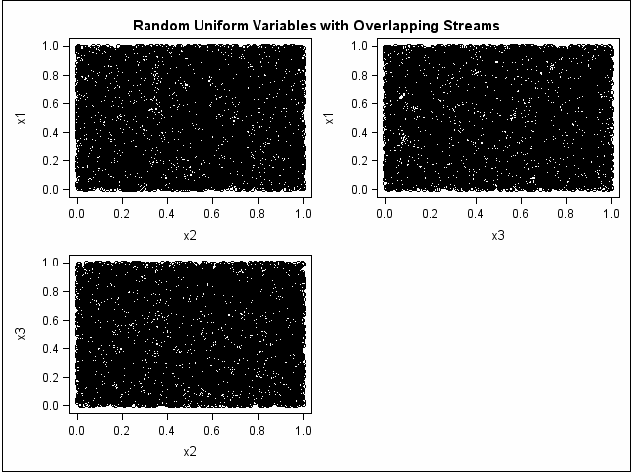Random Uniform Variables with Overlapping Streams: Plot 2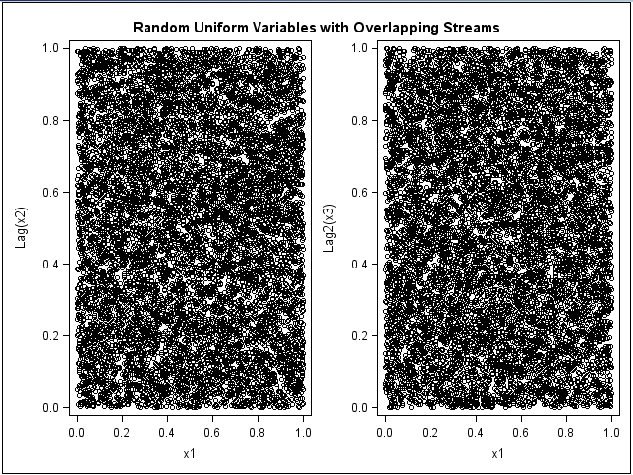In Example: Generating Random Uniform Variables with Overlapping Streams, it appears that the variables are independent. However, even this programming approach might not work well in general. The random-number functions and CALL routines have a period of only 231 - 2 or less (approximately 2.1 billion). When this limit is reached, the stream repeats. Modern computers performing complicated simulations can easily exhaust the entire stream in minutes.

A better approach to generating random uniform variables is to use the RAND function, where multiple streams are not permitted. The RAND function has a period of 219937 - 1. This limit will never be reached, at least with computers of the early 21st century. The number 219937 - 1 is approximately 106000 (1 followed by 6000 zeros). In comparison, the largest value that can be represented in eight bytes on most computers that run SAS is approximately 10307.

The RAND function, which is the latest random-number function that was designed, does not allow multiple streams. The RAND function uses a different algorithm from the random-number CALL routines, which allow you to create multiple streams with multiple seeds. Because the state of the RAND process cannot be captured by a single seed, you cannot stop and restart the generator from its stopping point. Therefore, the RAND function allows only a single stream of numbers, but it can be used to make multiple streams, just as the RANUNI function can.

### Starting, Stopping, and Restarting a Stream

A reasonable use of the random-number CALL routines is starting and stopping a single stream, provided the stream never exhausts the RANUNI stream. For example, you might want SAS to perform iterations, stop, evaluate the results, and then restart the stream at the point it stopped. The following example illustrates this principle.

### Example: Starting, Stopping, and Restarting a Stream

This example generates a stream of five numbers, stops, restarts, generates five more numbers from the same stream, combines the results, and generates the full stream for comparison. In the first DATA step, the state of the random-number seed is stored in a macro variable seed for use as the starting seed in the next step. The separate streams in the example output match the full stream.

```options pageno=1 nodate ls=80 ps=64;

data u1(keep=x);
seed = 104;
do i = 1 to 5;
call ranuni(seed, x);
output;
end;
call symputx('seed', seed);
run;

data u2(keep=x);
seed = &seed;
do i = 1 to 5;
call ranuni(seed, x);
output;
end;
run;

data all;
set u1 u2;
z = ranuni(104);
run;

proc print label;
title 'Random Uniform Variables with Overlapping Streams';
label x = 'Separate Streams' z = 'Single Stream';
run;```

```               Random Uniform Variables with Overlapping Streams               1

Separate     Single
Obs     Streams     Stream

1     0.23611    0.23611
2     0.88923    0.88923
3     0.58173    0.58173
4     0.97746    0.97746
5     0.84667    0.84667
6     0.80484    0.80484
7     0.46983    0.46983
8     0.29594    0.29594
9     0.17858    0.17858
10     0.92292    0.92292
```

### Example: Changing Seeds in a CALL Routine and in a Function

If you use a CALL routine to change the seed, the results are different from using a function to change the seed. The following example shows the difference.

```data seeds;
retain Seed1 Seed2 Seed3 104;
do i = 1 to 10;
call ranuni(Seed1,X1);
call ranuni(Seed2,X2);
X3 = ranuni(Seed3);
if i = 5 then do;
Seed2 = 17;
Seed3 = 17;
end;
output;
end;
run;

proc print data = seeds;
title 'Random Uniform Variables with Overlapping Streams';
id i;
run;```

```               Random Uniform Variables with Overlapping Streams               3

i       Seed1           Seed2    Seed3       X1         X2         X3

1     507036483     507036483     104     0.23611    0.23611    0.23611
2    1909599212    1909599212     104     0.88923    0.88923    0.88923
3    1249251009    1249251009     104     0.58173    0.58173    0.58173
4    2099077474    2099077474     104     0.97746    0.97746    0.97746
5    1818205895            17      17     0.84667    0.84667    0.84667
6    1728390132     310018657      17     0.80484    0.14436    0.80484
7    1008960848    1055505749      17     0.46983    0.49151    0.46983
8     635524535    1711572821      17     0.29594    0.79701    0.29594
9     383494893     879989345      17     0.17858    0.40978    0.17858
10    1981958542    1432895200      17     0.92292    0.66724    0.92292
```

Changing Seed2 in the CALL RANUNI statement when i=5, forces the stream for X2 to deviate from the stream for X1. However, changing Seed3 in the RANUNI function has no effect. The X3 stream continues on as if nothing has changed, and the X1 and X3 streams are the same.Previous Page | Next Page | Top of Page